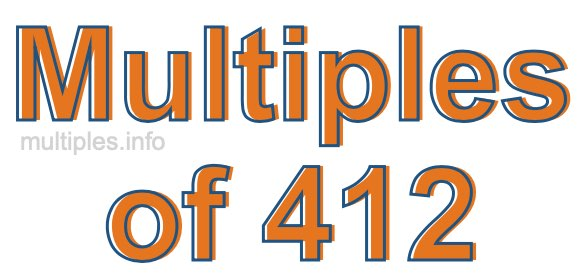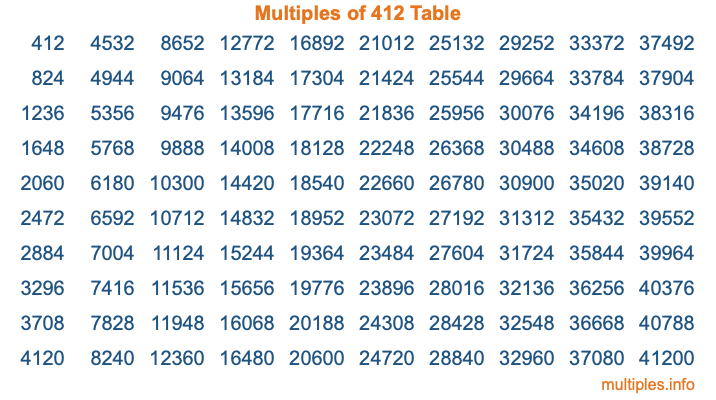Multiples of 412Welcome to the Multiples of 412 page. Here we will first teach you everything you will ever need to know about the multiples of 412, and then give you a study guide summary of everything we taught you to make sure you remember it all. Use this page to look up facts and learn information about the multiples of 412. This page will make you a multiples of four hundred twelve expert!

Definition of Multiples of 412
Multiples of 412 are all the numbers that when divided by 412 equal an integer. Each of the multiples of 412 are called a multiple. A multiple of 412 is created by multiplying 412 by an integer.

Therefore, to create a list of multiples of 412, you start with 1 multiplied by 412, then 2 multiplied by 412, then 3 multiplied by 412, and so on for as long as you want. Thus, the list of the first five multiples of 412 is 412, 824, 1236, 1648, and 2060. To see a larger list of multiples of 412, see the printable image of Multiples of 412 further down on this page. We also have a category where you can choose any nth multiple of 412.

Multiples of 412 Checker
The Multiples of 412 Checker below checks to see if any number of your choice is a multiple of 412. In other words, it checks to see if there is any number (integer) that when multiplied by 412 will equal your number. To do that, we divide your number by 412. If the the quotient is an integer, then your number is a multiple of 412.

Is  a multiple of 412?

Least Common Multiple of 412 and ...
A Least Common Multiple (LCM) is the lowest multiple that two or more numbers have in common. This is also called the smallest common multiple or lowest common multiple and is useful to know when you are adding our subtracting fractions. Enter one or more numbers below (412 is already entered) to find the LCM.

Check out our LCM Calculator if you need more details about the Least Common Multiple or if you need the LCM for different numbers for adding and subtraction fractions.

nth Multiple of 412
As we stated above, 412 is the first multiple of 412, 824 is the second multiple of 412, 1236 is the third multiple of 412, and so on. Enter a number below to find the nth multiple of 412.

th multiple of 412

Multiples of 412 vs Factors of 412
412 is a multiple of 412 and a factor of 412, but that is where the similarities end. All postive multiples of 412 are 412 or greater than 412. All positive factors of 412 are 412 or less than 412.

Below is the beginning list of multiples of 412 and the factors of 412 so you can compare:

Multiples of 412: 412, 824, 1236, 1648, 2060, etc.

Factors of 412: 1, 2, 4, 103, 206, 412

As you can see, the multiples of 412 are all the numbers that you can divide by 412 to get a whole number. The factors of 412, on the other hand, are all the whole numbers that you can multiply by another whole number to get 412.

It's also interesting to note that if a number (x) is a factor of 412, then 412 will also be a multiple of that number (x).

Multiples of 412 vs Divisors of 412
The divisors of 412 are all the integers that 412 can be divided by evenly. Below is a list of the divisors of 412.

Divisors of 412: 1, 2, 4, 103, 206, 412

The interesting thing to note here is that if you take any multiple of 412 and divide it by a divisor of 412, you will see that the quotient is an integer.

Multiples of 412 Table
Below is an image of the first 100 multiples of 412 in a table. The table is in chronological order, column by column. The first column has the first ten multiples of 412, the second column has the next ten multiples of 412, and so on.The Multiples of 412 Table is also referred to as the 412 Times Table or Times Table of 412. You are welcome to print out our table for your studies.

Negative Multiples of 412
Although not often discussed or needed in math, it is worth mentioning that you can make a list of negative multiples of 412 by multiplying 412 by -1, then by -2, then by -3, and so on, to get the following list of negative multiples of 412:

-412, -824, -1236, -1648, -2060, etc.

Multiples of 412 Summary
Below is a summary of important Multiples of 412 facts that we have discussed on this page. To retain the knowledge on this page, we recommend that you read through the summary and explain to yourself or a study partner why they hold true.

There are an infinite number of multiples of 412.

A multiple of 412 divided by 412 will equal a whole number.

412 divided by a factor of 412 equals a divisor of 412.

The nth multiple of 412 is n times 412.

The largest factor of 412 is equal to the first positive multiple of 412.

412 is a multiple of every factor of 412.

412 is a multiple of 412.

A multiple of 412 divided by a divisor of 412 equals an integer.

412 divided by a divisor of 412 equals a factor of 412.

Any integer times 412 will equal a multiple of 412.

Multiples of a Number
Here you can get the multiples of another number, all with the same attention to detail as we did for multiples of 412 on this page.

Multiples of
Multiples of 413
Did you find our page about multiples of four hundred twelve educational? Do you want more knowledge? Check out the multiples of the next number on our list!